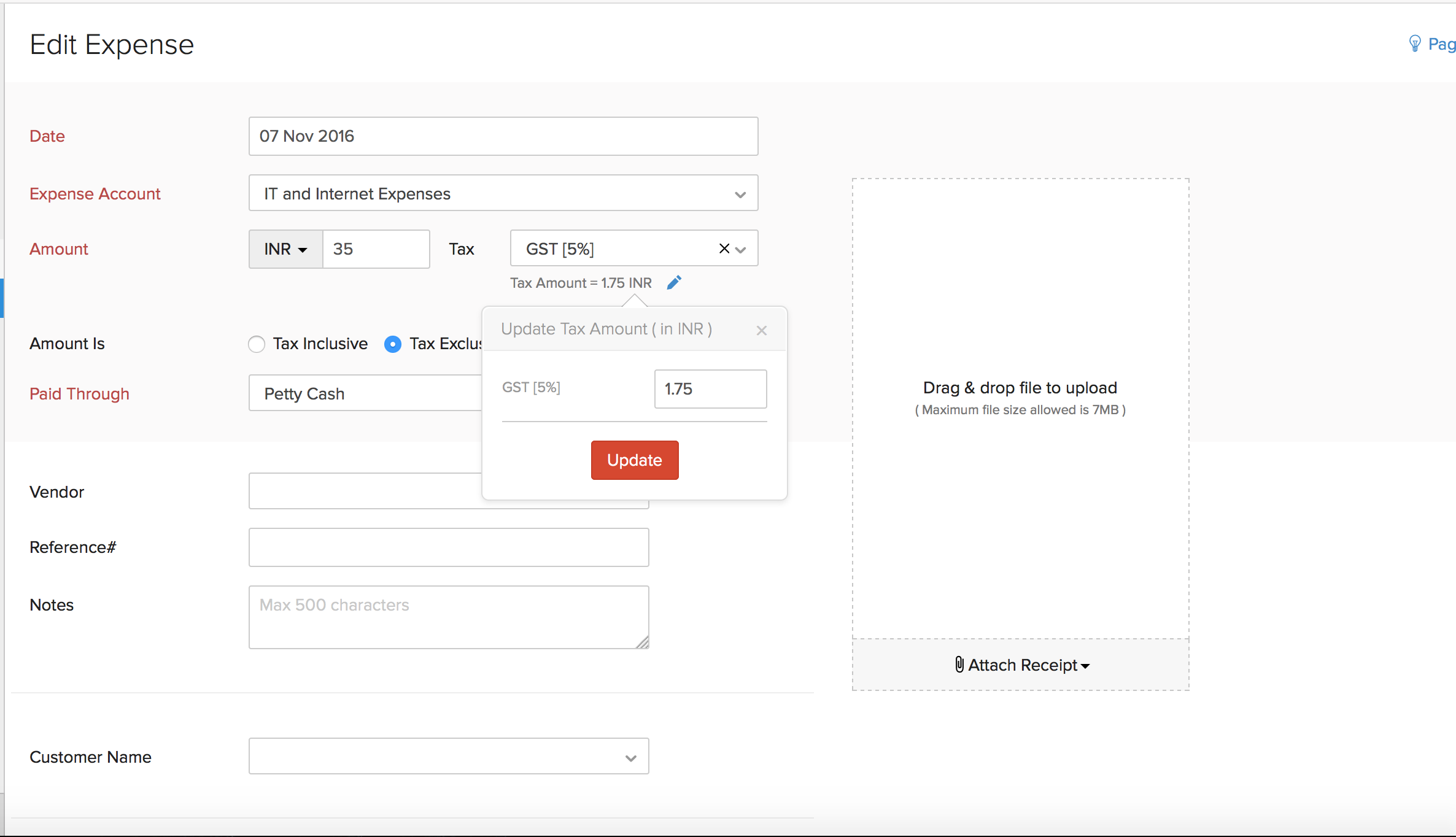# How do I manually change the tax amount in an expense?

There might be cases wherein the tax amount in an expense varies because your vendor calculates the tax applied on the transaction differently.

Zoho Books allows you to account for these minor variations by allowing you to manually change i.e over-ride an automatically calculated tax amount, once you apply a tax to a transaction.

Let’s take an example where you print two sets of flyers, A5 (100 Nos.) and A4 (50 Nos.) to give it out to customers at a Sales conference.

The receipt of the expense has the following details:

A5 Flyer (100 Nos.) - \$ 123.45

A4 Flyer (50 Nos. ) - \$ 123.46

Sub Total - \$ 246.91

The vendor also adds a 10% tax for every item in the bill.

10% of 123.45 = 12.35 (Rounded to 2 decimal places)

10% of 123.46 = 12.35 (Rounded to 2 decimal places)

Now tax amount will be 12.35+12.35 = \$24.70

Total - \$ 246.91 + \$ 24.70 = \$ 271.61

However, when you attach the receipt and add the expense details in Zoho Books, you will enter the sub total of \$ 246.91 and you will choose the tax of 10% from the drop-down (or create a new one) and mark it as Tax Exclusive.

Now, the tax in Zoho Books will be calculated for the whole amount (\$ 246.91) rather than how it was done by the vendor (apply tax to each item). This would result in a tax amount of \$24.69.

From the above calculation you can observe a small variation between the calculations done by the vendor and Zoho Books.

Under such cases, you can manually adjust these variations by clicking on the Change option next to the Tax Amount and modify the tax amount that has been calculated automatically.Finally, click on the Save button to save the transaction with the modified tax rate.YesNoThank you for your feedback!